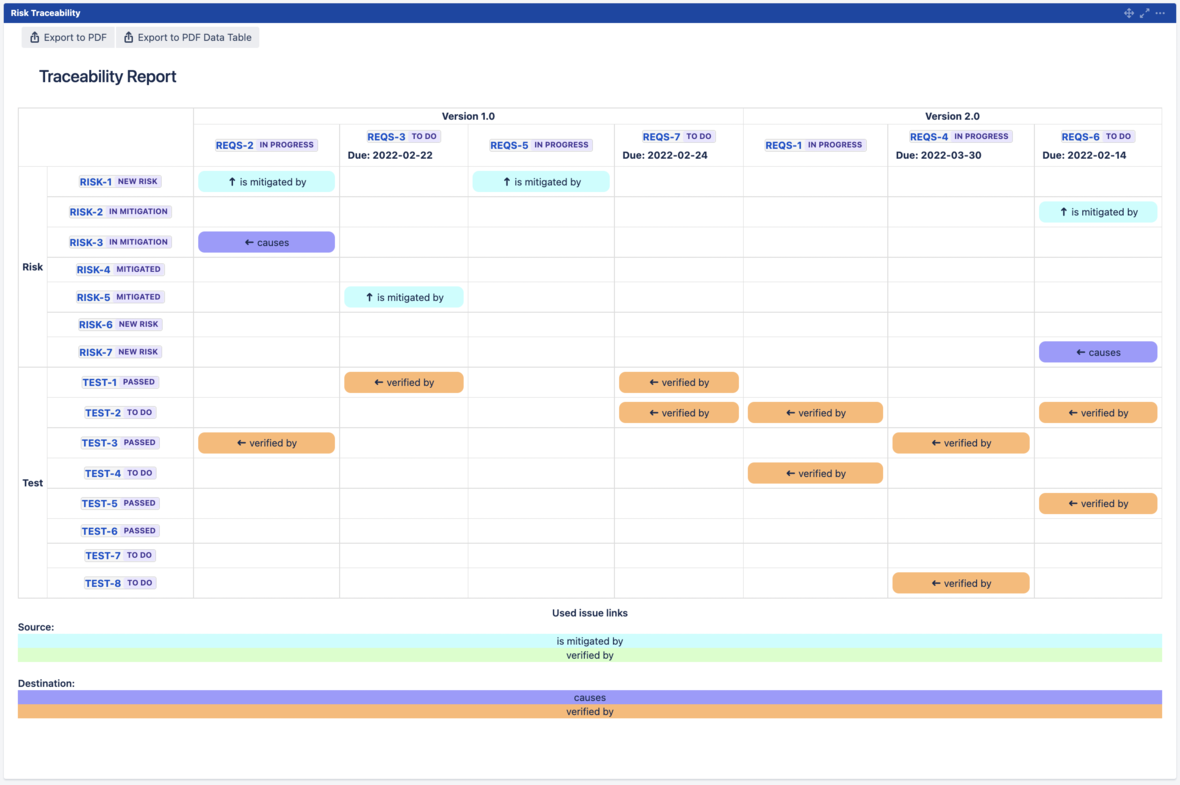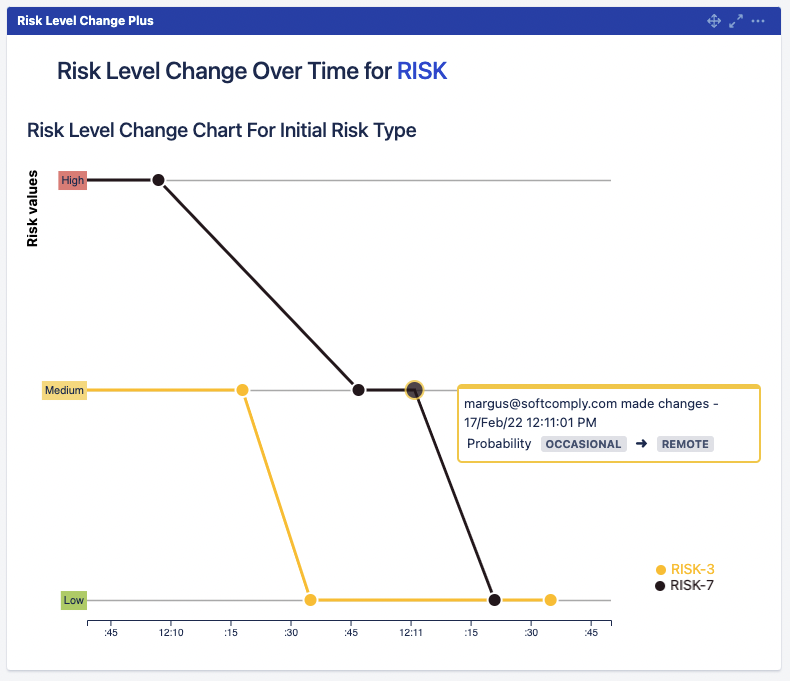Like   Tweet   in

## What's New in February 2022

 /* styles */ In this February newsletter we will provide you with an overview of the brand new risk reports of the SoftComply Risk Manager and the Risk Manager Plus apps on Jira Server and Data Center.
 table div table+table+table+table div table{width:100%;padding:0}table div table+table+table+table div table img{width:96.23%;padding:0;float:none}table div table+table+table+table div table td{width:100%;padding:0 1.88% 18px}/* styles */## NEW RISK REPORTS IN SERVER & DATA CENTER

 table div table+table+table+table+table+table+table div table{width:100%;padding:0}table div table+table+table+table+table+table+table div table img{width:96.23%;padding:0;float:none}table div table+table+table+table+table+table+table div table td{width:100%;padding:0 1.88% 18px}/* styles */## TRACEABILITY REPORT

 /* styles */ Risk Traceability report is provided in the format of a two-dimensional traceability matrix displaying the link type (e.g. “is mitigated by”) and the direction (small arrow pointing to the target) between risks and other Jira issues of your choice. The matrix is fully customisable and the user can specify the risks and Jira issues to be mapped as well as the link types to be displayed. To learn more about the traceability report, please continue reading the full post here.Risk Traceability Report in Jira Dashboard
 table div table+table+table+table+table+table+table+table+table+table+table div table{width:100%;padding:0}table div table+table+table+table+table+table+table+table+table+table+table div table img{width:96.23%;padding:0;float:none}table div table+table+table+table+table+table+table+table+table+table+table div table td{width:100%;padding:0 1.88% 18px}/* styles */## RISK HISTORY REPORT

 /* styles */ Risk History report is a graphical presentation of changes made to the risk parameters like Severity and Probability that impact the overall Risk Class. Each point of change in the history of a risk highlights what changes were made, who made them and when. To learn more about the risk history report, please continue reading the full post here.Risk History of multiple risks in Jira Dashboard
 table div table+table+table+table+table+table+table+table+table+table+table+table+table+table+table div table{width:100%;padding:0}table div table+table+table+table+table+table+table+table+table+table+table+table+table+table+table div table img{width:96.23%;padding:0;float:none}table div table+table+table+table+table+table+table+table+table+table+table+table+table+table+table div table td{width:100%;padding:0 1.88% 18px}/* styles */## RISK MATRIX REPORT

 /* styles */ Risk Matrix report is fully customisable – users can choose which risks to display from which project and whether to display only the Initial Risk Matrix, the Residual Risk Matrix or both together. To learn more about the risk matrix report, please continue reading the full post here.
 table div table+table+table+table+table+table+table+table+table+table+table+table+table+table+table+table+table+table div table td,table.module-17{width:100%;padding:0}table div table+table+table+table+table+table+table+table+table+table+table+table+table+table+table+table+table+table div table{width:100%;float:none;margin-left:auto;margin-right:auto;padding:0}table div table+table+table+table+table+table+table+table+table+table+table+table+table+table+table+table+table+table div table a{border:0 none;text-decoration:none}table div table+table+table+table+table+table+table+table+table+table+table+table+table+table+table+table+table+table div table img{width:100%!important;border:0 none;text-decoration:none}/* styles */
 table div table+table+table+table+table+table+table+table+table+table+table+table+table+table+table+table+table+table+table div table{width:100%;padding:0}table div table+table+table+table+table+table+table+table+table+table+table+table+table+table+table+table+table+table+table div table img{width:96.23%;padding:0;float:none}table div table+table+table+table+table+table+table+table+table+table+table+table+table+table+table+table+table+table+table div table td{width:100%;padding:0 1.88% 18px}/* styles */## RISK TABLE REPORT

 /* styles */ Risk Table Report visualises user-specified risks in a spreadsheet view. Risk Table Report can be accessed either in Jira Dashboard or as a custom Jira report. To learn more about the risk table report, please continue reading the full post here.
 table div table+table+table+table+table+table+table+table+table+table+table+table+table+table+table+table+table+table+table+table+table+table+table div table{width:100%;padding:0}table div table+table+table+table+table+table+table+table+table+table+table+table+table+table+table+table+table+table+table+table+table+table+table div table img{width:96.23%;padding:0;float:none}table div table+table+table+table+table+table+table+table+table+table+table+table+table+table+table+table+table+table+table+table+table+table+table div table td{width:100%;padding:0 1.88% 18px}/* styles *//* styles */ For more details on the Risk Reports of the SoftComply Risk Manager and the Risk Manager Plus on Jira Server and Data Center, please see our latest blog post on our website.
 table div table+table+table+table+table+table+table+table+table+table+table+table+table+table+table+table+table+table+table+table+table+table+table+table+table div table{width:100%;padding:0}table div table+table+table+table+table+table+table+table+table+table+table+table+table+table+table+table+table+table+table+table+table+table+table+table+table div table img{width:96.23%;padding:0;float:none}table div table+table+table+table+table+table+table+table+table+table+table+table+table+table+table+table+table+table+table+table+table+table+table+table+table div table td{width:100%;padding:0 1.88% 18px}/* styles */table div table+table+table+table+table+table+table+table+table+table+table+table+table+table+table+table+table+table+table+table+table+table+table+table+table+table div table{width:100%;padding:0}table div table+table+table+table+table+table+table+table+table+table+table+table+table+table+table+table+table+table+table+table+table+table+table+table+table+table div table img{width:96.23%;padding:0;float:none}table div table+table+table+table+table+table+table+table+table+table+table+table+table+table+table+table+table+table+table+table+table+table+table+table+table+table div table td{width:100%;padding:0 1.88% 18px}/* styles */## NEW YOUTUBE PLAYLIST ON eQMS SOLUTION ON CONFLUENCE

In case you cannot join the webinars, feel free to check out the webinar recordings in our YouTube channel about:

▪ Medical Device Regulations & the FDA compliant eQMS Solution in Confluence Playlist,
▪ Risk Management in Jira with the SoftComply Risk Manager app video,
▪ Establishing Risk Traceability in Jira and Confluence Playlist.
 ▪ Medical Device Regulations & the FDA compliant eQMS Solution in Confluence Playlist,
 ▪ Risk Management in Jira with the SoftComply Risk Manager app video,
 ▪ Establishing Risk Traceability in Jira and Confluence Playlist.
 table div table+table+table+table+table+table+table+table+table+table+table+table+table+table+table+table+table+table+table+table+table+table+table+table+table+table+table+table+table div table td,table.module-28{width:100%;padding:0}table div table+table+table+table+table+table+table+table+table+table+table+table+table+table+table+table+table+table+table+table+table+table+table+table+table+table+table+table+table div table{width:100%;float:none;margin-left:auto;margin-right:auto;padding:0}table div table+table+table+table+table+table+table+table+table+table+table+table+table+table+table+table+table+table+table+table+table+table+table+table+table+table+table+table+table div table a{border:0 none;text-decoration:none}table div table+table+table+table+table+table+table+table+table+table+table+table+table+table+table+table+table+table+table+table+table+table+table+table+table+table+table+table+table div table img{width:100%!important;border:0 none;text-decoration:none}/* styles */
 table div table+table+table+table+table+table+table+table+table+table+table+table+table+table+table+table+table+table+table+table+table+table+table+table+table+table+table+table+table+table div table{width:100%;padding:0}table div table+table+table+table+table+table+table+table+table+table+table+table+table+table+table+table+table+table+table+table+table+table+table+table+table+table+table+table+table+table div table img{width:96.23%;padding:0;float:none}table div table+table+table+table+table+table+table+table+table+table+table+table+table+table+table+table+table+table+table+table+table+table+table+table+table+table+table+table+table+table div table td{width:100%;padding:0 1.88% 18px}/* styles */Benefits of the SoftComply Static Snapshots for Confluence Cloud:

▪ Capture and Freeze Data in Confluence
▪ Display Static Data in Confluence pages
▪ Approve Confluence pages that do not change
▪ Create Jira macros & freeze the Jira data with the Snapshot
 ▪ Capture and Freeze Data in Confluence
 ▪ Display Static Data in Confluence pages
 ▪ Approve Confluence pages that do not change
 ▪ Create Jira macros & freeze the Jira data with the Snapshot
 table div table+table+table+table+table+table+table+table+table+table+table+table+table+table+table+table+table+table+table+table+table+table+table+table+table+table+table+table+table+table+table+table div table{width:100%;padding:0}table div table+table+table+table+table+table+table+table+table+table+table+table+table+table+table+table+table+table+table+table+table+table+table+table+table+table+table+table+table+table+table+table div table img{width:96.23%;padding:0;float:none}table div table+table+table+table+table+table+table+table+table+table+table+table+table+table+table+table+table+table+table+table+table+table+table+table+table+table+table+table+table+table+table+table div table td{width:100%;padding:0 1.88% 18px}/* styles */Benefits of the SoftComply Risk Manager PLUS for Jira Server & Data Center:

▪ Supports Hazard Analysis and FMEA
▪ Supports FMEA with Detectability
▪ Displays risks in Matrices & RPN
▪ Supports FDA approval and CE-marking process
▪ Targeted towards safety critical domain users
 ▪ Supports Hazard Analysis and FMEA
 ▪ Supports FMEA with Detectability
 ▪ Displays risks in Matrices & RPN
 ▪ Supports FDA approval and CE-marking process
 ▪ Targeted towards safety critical domain users
 /* styles */ For full list of features of SoftComply Risk Manager PLUS, please check out the User Guide. Try it out for free for a month and/or contact us to get a demo & discussion about the features.
 table div table+table+table+table+table+table+table+table+table+table+table+table+table+table+table+table+table+table+table+table+table+table+table+table+table+table+table+table+table+table+table+table+table+table+table div table{width:100%;padding:0}table div table+table+table+table+table+table+table+table+table+table+table+table+table+table+table+table+table+table+table+table+table+table+table+table+table+table+table+table+table+table+table+table+table+table+table div table img{width:96.23%;padding:0;float:none}table div table+table+table+table+table+table+table+table+table+table+table+table+table+table+table+table+table+table+table+table+table+table+table+table+table+table+table+table+table+table+table+table+table+table+table div table td{width:100%;padding:0 1.88% 18px}/* styles */Benefits of the SoftComply eQMS:

▪ Full Quality Management System for medical device co-s
▪ On ISO13485, IEC62304, ISO14971, FDA 21CFR 820
▪ Fully customizable with embedded guidance
▪ Reduces the cost of compliance
▪ Most competitive price
 ▪ Full Quality Management System for medical device co-s
 ▪ On ISO13485, IEC62304, ISO14971, FDA 21CFR 820
 ▪ Fully customizable with embedded guidance
 ▪ Reduces the cost of compliance
 ▪ Most competitive price
 /* styles */ SoftComply eQMS on Confluence Cloud: Contact us for a demo call of the FDA 21 CFR 11 compliant eQMS Solution on Confluence Cloud.
 table div table+table+table+table+table+table+table+table+table+table+table+table+table+table+table+table+table+table+table+table+table+table+table+table+table+table+table+table+table+table+table+table+table+table+table+table+table+table div table{width:100%;padding:0}table div table+table+table+table+table+table+table+table+table+table+table+table+table+table+table+table+table+table+table+table+table+table+table+table+table+table+table+table+table+table+table+table+table+table+table+table+table+table div table img{width:96.23%;padding:0;float:none}table div table+table+table+table+table+table+table+table+table+table+table+table+table+table+table+table+table+table+table+table+table+table+table+table+table+table+table+table+table+table+table+table+table+table+table+table+table+table div table td{width:100%;padding:0 1.88% 18px}/* styles */Benefits of the SoftComply Risk Manager:

▪ Supports any software system/product risk management
▪ For both Jira Server & Cloud users
▪ Fully customizable for any type of risk project
▪ Automated traceability
▪ Support software system/product risk management
▪ Most competitive price
 ▪ Supports any software system/product risk management
 ▪ For both Jira Server & Cloud users
 ▪ Fully customizable for any type of risk project
 ▪ Automated traceability
 ▪ Support software system/product risk management
 ▪ Most competitive price
 /* styles */ For full list of features of SoftComply Risk Manager, please check out the User Guide. Try it out for free for a month and/or contact us to get a demo & discussion about the features.
 table div table+table+table+table+table+table+table+table+table+table+table+table+table+table+table+table+table+table+table+table+table+table+table+table+table+table+table+table+table+table+table+table+table+table+table+table+table+table+table+table+table div table{width:100%;padding:0}table div table+table+table+table+table+table+table+table+table+table+table+table+table+table+table+table+table+table+table+table+table+table+table+table+table+table+table+table+table+table+table+table+table+table+table+table+table+table+table+table+table div table img{width:96.23%;padding:0;float:none}table div table+table+table+table+table+table+table+table+table+table+table+table+table+table+table+table+table+table+table+table+table+table+table+table+table+table+table+table+table+table+table+table+table+table+table+table+table+table+table+table+table div table td{width:100%;padding:0 1.88% 18px}/* styles */